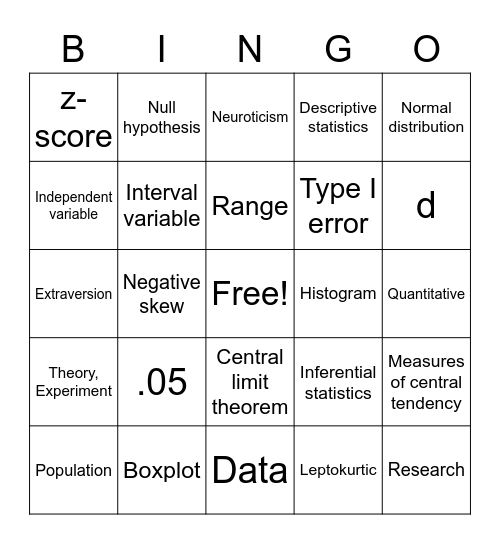# Statistics BingoThis bingo card has a free space and 47 words: Statistics, Research, Data science, Data, .05, Hypothesis, Null hypothesis, Variables, Independent variable, Nominal variable, Interval variable, Descriptive statistics, Measures of central tendency, Inferential statistics, Median, Mean, Range, Histogram, Modality, Normal distribution, Negative skew, Leptokurtic, z-score, Type I error, Type II error, d, Population, Sample, Central limit theorem, Theory, Experiment, Alternative hypothesis, Significance, p-value, Histogram, Misleading, Boxplot, Quantitative, Confirmatory, Independent t-test, Dependent design, One sample t-test, Neuroticism, Extraversion, Test statistic, Degrees of freedom, Effect size and Assumptions.

⚠ This card has duplicate items: Histogram (2)

## Play Online